Supervised Machine Learning with Python Scikit Learn(sklearn) in Four Lines of Code

Machine Learning(ML) consists of developing a mathematical model from an experimental dataset. Three techniques are used to create a model: supervised learning, unsupervised learning, and reinforcement learning.

This article focuses on supervised learning, which is the most common technique. In supervised learning, a machine receives data characterized by variables x and annotated by variable y. We call variable x the features in the machine learning vocabulary, while the variable y is called a label. The goal of supervised ML is for the machine to learn to predict the value of y based on the features x that are given to it. That is why y is also referred to as the target variable.

To train the machine or for the machine to learn, we begin by giving the machine lot of data(dataset). Then we specify the type of model that the machine has to learn and set the model’s hyperparameters. There are several models to choose from — for example, linear model, polynomial model, decision tree, or a neural network. Once we have selected our model, we also specify the hyperparameters of our model. For example, we set the number of branches in a decision tree and the neurons in our neural network. Once we finish the hyperparameter selection, the machine starts learning. In the learning process, the machine uses an optimization algorithm to find the model’s parameters that give the best performance for the given dataset (this is the training phase). Once the training phase is complete, our machine learning model is ready to be used. When the machine receives new data without a label(y), it uses the model to predict the value of y.

We can use the supervised ML technique to solve regression problems when y is a continuous variable(quantitative). We can also use supervised learning to solve classification problems when y is a discrete variable(qualitative).

Scikit Learn

Scikit learn renders machine learning efficient and straightforward. All the models and machine learning algorithms have already been implemented with object-oriented architecture, where each model has its class. To create a model, we generate an object of the corresponding class to that model. This object of the corresponding class is what is called an estimator in Scikit Learn. We can also indicate in parentheses the hyperparameters of our model. See equation 1

First step in Scikit learn is to select an estimator and indicate its hyperparameters

object = estimator(hyperparameters) — — — -equation 1

Example: model = LinearRegression( — — — -)

For example, in gradient descent, we can indicate the learning rate as the hyperparameter as follows:

model = SGDRegressor(eta0 = 0.2) # learning_rate = 0.2

In a Random Forest, we can set the number of trees as hyperparameters as follows

model = RandomForestClassifier(n_estimators=100) # the number of trees = 100

The first step that we just described constitutes the initialization of our model. Once the model has been initialized, we can then train, evaluate and use our model by using three methods that we find in all the classes of Scikit learn. Having three techniques found in all sklearn classes is very helpful and makes the different classes easier to use.

We have so many models or classes in sklearn. For example, Linear regression, Decision Tree, Random Forest, K-NN, SVM, Neural Network, etc. These models have different mechanisms, but the user interface is the SAME.

model interface = fit, score, predict for all the models.

Having the same interface means that we will write similar code if we want to develop a model such as linear regression, decision tree, etc. For example,

To develop a Linear Regression model, we will have the following four lines of code:

model = LinearRegression( )

model.fit(X, y)

model.score(X, y)

model.predict(X)

To develop a Decision Tree model, we will have a similar code:

model = DecisionTreeClassifier( )

model.fit(X, y)

model.score(X, y)

model.predict(X)

To develop a Support Vector Machine, we use:

model = SVC( )

model.fit(X, y)

model.score(X, y)

model.predict(X)

To develop a Random Forest model, we use:

model = RandomForestClassifier( )

model.fit(X, y)

model.score(X, y)

model.predict(X)

It should be noted that the hyperparameters for each model are different and have to be set.

The second step in sklearn supervised learning is to train the model on the datasets X and y.

In general, to train our model, we use the “fit” method ( model.fit(X, y) ) in which we can pass our data, X, y. X and y are presented in 2 different NumPy tables, Feature Matrix and Target Vector:

The Feature Matrix(x) consists of 2 dimensions, n_samples, and n_features.

The first dimension in the feature matrix is the number of samples (n_samples) present in our dataset, and the second dimension is the number of features (n_features) in our dataset. The target Vector (y) consists of n_samples. The target vector is the number of targets and not the number of features.

Third step: we evaluate our model using the score method (model.score(X, y)).

Once our model is trained, we can evaluate it using the “model.score” method. In this method, we pass X and y into the model, and the machine uses the X data to make a prediction and compares this prediction with the y value present in the data. Thus, the performance of the model depends on how well the model predicts y.

The fourth step in our SKlearn supervised learning

Once we are satisfied with the model’s performance, we can use it to make new predictions. To make new predictions, we use the predict method (model.predict(X)).

Thus, these are the four lines of code that can be used to develop a machine learning model with sklearn.

model = estimator(hyperparameters )

model.fit(X, y)

model.score(X, y)

model.predict(X)

There are various modules in sklearn. Each module has several models. For example, the module sklearn.SVM: Support Vector Machine consists of estimators or models such as SVC, SVR, etc.

To use these algorithms, we import the corresponding module. For example, for linear regression, we import the LinearRegression model from sklearn.linear_model as follow:

from sklearn.linear_model import LinearRegression

# In the above code, we import the LinearRegression model from sklearn.linear_model module. If we import sklearn only, the program will not work.

Here is how I use sklearn to solve regression and classification problems.

--

--

--

## More from Celestin Ntemngwa

Love podcasts or audiobooks? Learn on the go with our new app.

## Guiding Self -Driving Car Using Behavioral Cloning## Semantic Segmentation in Seismic Images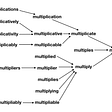## Understanding Dimensionality Reduction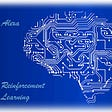## Neural Physics Attempt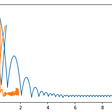## How to get the team started with AI/ Machine Learning?## Gender and Race Change on Your Selfie with Neural Nets## Decentralized Machine Learning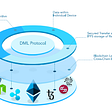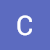## Difference Between Data Science And Machine Learning With Python?## Create Custom Datasets within Seconds